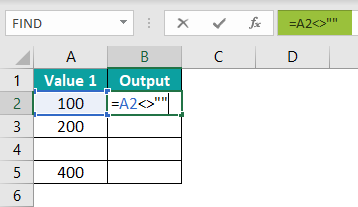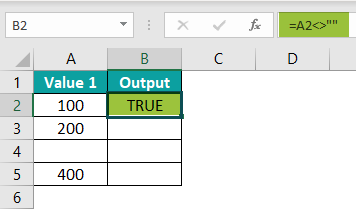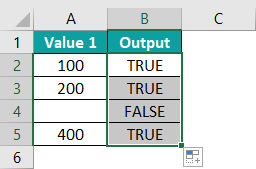# Not Equal To In ExcelArticle byExcelMojo TeamThe Not Equal to in Excel is a feature to compare two numerical values or two Text values. The Sign or Symbol for Not Equal to is <>”. The Not Equal to in Excel returns “True and False” as the output.

• True denotes that the compared values are different or not equal.
• False denotes that the compared values are the same or equal.

We do not have a pre-defined formula in Excel. However, we can manually write the function as “=value1<>value2”, where Value1 and Value2 are the Excel cell references or the numeric and text values. For example, the image below shows the numerical values in columns A and B. Here, we will compare the numbers in the columns using the Not Equal to in Excel.

Enter the formula =A2<>B2 in cell C2, press the “Enter” key, and drag the formula using the fill handle to cell C3.

The output in the example is TRUE in cell C2 and FALSE in cell C3, as shown below.

###### Key Takeaways
• The Not Equal to in Excel checks whether the values getting compared, numerical or text, are equal or not.
• The function always requires two values to check. While comparing values, if one of the values is a blank cell, we will get the output as “True”. And if both the cells are blank, we get the output as “False”. These are a few scenarios of the Boolean default values.
• We can use conditional functions such as the IF function, COUNTIF function, and the SUMIF function to get an alternate output instead of the default “True” or “False”.

### The Symbol For “Not Equal To” in Excel

The Symbol for Not Equal to in Excel is “<>”. [The less than symbol “<” and the greater than symbol “>” facing each other].

### How Is The “Not Equal To” Sign Used In Excel?

We can use the Not Equal to in Excel as follows:

• Compare Two Numeric Values.
• Compare Two Text Values.
• Compare using the IF Function With a “Not Equal To” Condition.
• Compare using the COUNTIF Function With a “Not Equal To” Condition.
• Compare using the SUMIF Function With a “Not Equal To” Condition.

#### #1 – Compare Two Numeric Values

We will Compare Two Numeric Values using the Not Equal to in Excel.

In the following table, the data is,

• Column A contains Value1.
• Column B contains Value2.
• Column C is for the Output.

The steps to Compare Two Numeric Values are as follows:

Step 1: Select cell C2 and enter the formula =A2<>B2.

Step 2: Press the “Enter” key. The result is “True”.

Step 3: Drag the formula from cell C2 to cell C5. The output is shown in the image below.

#### #2 – Compare Two Text Values

We will Compare Two Text Values using the Not Equal to in Excel.

In the following table, the data is,

• Column A contains Text1.
• Column B contains Text2.
• Column C is for the Output.

The steps to Compare Two Text Values are as follows:

Step 1: Select cell C2 and enter the formula =A2<>B2.

Step 2: Press the “Enter” key. The result is “True”.

Step 3: Drag the formula from cell C2 to cell C5. The output is shown in the image below.

#### #3 – Use Of IF Function With “Not Equal To” Condition

We will compare values using the IF Excel function With “Not Equal To” Condition. The IF function permits us to get any desired output in place of the default “True or False”.

In the following table, the data is,

• Column A contains the Fruits.
• Column B contains the Status.
• Column C for the output.

The steps to compare cells using the IF function are as follows:

Step 1:  Select cell C2 and enter the formula =IF(B2<>“Order,” “Sale,” “Out of stock”).

Step 2: Press the “Enter” key. The result is “Out of Stock” w.r.t the IF function condition.

Step 3: Now, drag the formula from cell C2 to cell C5. The output is shown in the image below.

#### #4 – COUNTIF Function With “Not Equal To” Condition

The COUNTIF function is from the COUNT function family in Excel, like the COUNTA and COUNTBLANK functions. It is a pre-defined function in Excel that counts all the cells in the range as specified by the conditions.

The formula of the COUNTIF function is:

=COUNTIF(range,criteria)

We will compare values using the COUNTIF Function With “Not Equal To” Condition.

In the following table, the Pokémon data is,

• Column A shows the Name.
• Column B contains the Type.
• Column C contains the Total Status.
• Columns E and F are for the COUNTIF

The steps to compare cells using the COUNTIF function are as follows:

Step 1:  Select cell F2 and enter the formula =COUNTIF(B2:B7,“<>Fighter”).

Step 2: Press the “Enter” key. The result is ‘4’ w.r.t the COUNTIF function condition.

Therefore, the output is 4, which denotes that there are 4 Pokémon that are not the “Fighter” type.

#### #5 – SUMIF Function With “Not Equal To” Condition

The SUMIF excel function is from the SUM function family in Excel, like the SUM and SUMPRODUCT functions. It is a pre-defined function in Excel that adds all the cells in the range as specified by the conditions.

The formula of the SUMIF function is,

=SUMIF(range,criteria,[sum_range])

We will compare values using the SUMIF Function With “Not Equal To” Condition.

In the following table, the data is,

• Column A shows the City.
• Column B contains the Category.
• Column C contains the Sates.
• Columns E, F, and G are for the Output.

The steps to compare cells using the SUMIF function are as follows:

Step 1:  Select cell G2 and enter the formula =SUMIF(C2:C7,“<>Snacks”).

Step 2: Press the “Enter” key. The result is ‘\$1,18,10,894’ w.r.t the SUMIF function condition.

### Important Things To Note

• The mathematical sign or symbol for Not Equal to is , however, in Excel, we use “<>” symbol. [The less than symbol “<” and the greater than symbol “>” facing each other].
• The Not Equal to in Excel is case-insensitive. So, whether the strings compared are uppercase, lowercase, or a combination of both, the function will return accurate results.
• We can also compare a numeric value with a text value, as shown below. Again, the output will be “True”, implying that the two values are different.

How do you write Not Equal To in Excel?

We write the Not Equal to in Excel as =value1<>value2 as we do not have an inbuilt function in Excel. [Value1 and Value2 are the cell references with numerical or text values].

What does “<>” mean in Excel?

The “<>” is the Not Equal to in Excel operator or symbol that detects whether the values match or not. If the values are the same, the output is “TRUE”, and if the values are different, the output is “False”.

How to write Not Equal To blank cells in Excel?

We will use the Not Equal to in Excel to compare with the blank cells.

In the following table, the data is,

1. Column A shows the Value.
2. Column B for the Output.

The steps to compare cells using Not Equal to in Excel are as follows:

Step 1:  Select cell B2 and enter the formula =A2<>“”.Step 2: Press the “Enter” key. The result is ‘True’.Step 3: Drag the formula from cell B2 to cell B5. The output is shown in the image below.